Win up to 100% scholarship on Aakash BYJU'S JEE/NEET courses with ABNAT Win up to 100% scholarship on Aakash BYJU'S JEE/NEET courses with ABNAT

# JEE Main 2022 June 24 – Shift 2 Physics Question Paper with Solutions

Candidates can download on this page the PDF version of the JEE Main 2022 June 24 – Shift 2 Physics Question Paper with Solutions. These answers were created by the JEE specialists at BYJU’S. Studying the JEE Main 2022 Question Papers will help candidates raise their JEE scores. Additionally, the candidate can efficiently prepare for the upcoming test by using the JEE Main 2022 answer key as a reference.
JEE Main 2022 June 24 – Shift 2 Physics Question Paper with Solutions

SECTION – A

Multiple Choice Questions: This section contains 20 multiple choice questions. Each question has 4 choices (1), (2), (3) and (4), out of which ONLY ONE is correct.

1. Identify the pair of physical quantities that have same dimensions :

(A) velocity gradient and decay constant

(B) wien’s constant and Stefan constant

(C) angular frequency and angular momentum

(D) wave number and Avogadro number

$$\begin{array}{l}=\frac{dv}{dx}\end{array}$$

⇒ Dimensions are

$$\begin{array}{l}\frac{\left [LT^{-1} \right ]}{\left [ L \right ]}=\left [ T^{-1} \right ]\end{array}$$

Decay constant λ has dimensions of [T–1] because of the relation

$$\begin{array}{l}\frac{dN}{dt}=-\lambda N\end{array}$$

⇒ Velocity gradient and decay constant have same dimensions.

2. The distance between Sun and Earth is R. The duration of year if the distance between Sun and Earth becomes 3R will be :

(A) √3 years

(B) 3 years

(C) 9 years

(D) 3√3 years

Sol. We know that

$$\begin{array}{l}T^{2}\propto R^3\end{array}$$
$$\begin{array}{l}\Rightarrow\left ( \frac{T’}{T} \right )^2=\left ( \frac{3R}{R} \right )^3\end{array}$$
$$\begin{array}{l}\Rightarrow \frac{T’}{T}=3\sqrt{3}\end{array}$$
$$\begin{array}{l}\Rightarrow T’=3\sqrt{3}\end{array}$$

3. A stone of mass m tied to a string is being whirled in a vertical circle with a uniform speed. The tension in the string is

(A) the same throughout the motion.

(B) minimum at the highest position of the circular path.

(C) minimum at the lowest position of the circular path.

(D) minimum when the rope is in the horizontal position.

Sol.At any θ:

$$\begin{array}{l}T-mg\ cos\theta=\frac{mv^2}{R}\end{array}$$
$$\begin{array}{l}\Rightarrow T=mg\ cos\theta+\frac{mv^2}{R}\end{array}$$

Since v is constant,

T will be minimum when cosθ is minimum.

⇒ θ = 180° corresponds to Tminimum.

4. Two identical charged particles each having a mass 10 g and charge 2.0 × 10–7 C are placed on a horizontal table with a separation of L between them such that they stay in limited equilibrium. If the coefficient of friction between each particle and the table is 0.25, find the value of L. [Use g = 10 ms–2]

(A) 12 cm

(B) 10 cm

(C) 8 cm

(D) 5 cm

Sol. According to given information:

$$\begin{array}{l}\frac{KQ^2}{L^2}=\mu mg\end{array}$$

Putting the values, we get

L = 12 cm

5. A Carnot engine takes 5000 kcal of heat from a reservoir at 727°C and gives heat to a sink at 127°C. The work done by the engine is

(A) 3 × 106 J

(B) Zero

(C) 12.6 × 106 J

(D) 8.4 × 106 J

Sol. Efficiency

$$\begin{array}{l}\eta=1-\frac{T_L}{T_H}\end{array}$$
$$\begin{array}{l}=1-\frac{400}{1000}\end{array}$$

= 0.6

$$\begin{array}{l}\Rightarrow 0.6=\frac{W}{Q}\end{array}$$

W = 0.6Q = 3000 kcal = 12.6 × 106 J

6. Two massless springs with spring constant 2 k and 9 k, carry 50 g and 100 g masses at their free ends. These two masses oscillate vertically such that their maximum velocities are equal. Then, the ratio of their respective amplitude will be

(A) 1 : 2

(B) 3 : 2

(C) 3 : 1

(D) 2 : 3

Sol.

$$\begin{array}{l}\omega_1 A_1=\omega_2 A_2\end{array}$$
$$\begin{array}{l}\Rightarrow \frac{A_1}{A_2}=\frac{\omega_2}{\omega_1}\end{array}$$
$$\begin{array}{l}=\sqrt{\frac{k_2}{m_2}}\times \sqrt{\frac{m_1}{k_1}}=\sqrt{\frac{9k}{100}\times\frac{50}{2k}}=\frac{3}{2}\end{array}$$

7. What will be the most suitable combination of three resistors A = 2 Ω, B = 4 Ω, C = 6 Ω so that (22/3)Ω is equivalent resistance of combination?

(A) Parallel combination of A and C connected in series with B.

(B) Parallel combination of A and B connected in series with C

(C) Series combination of A and C connected in parallel with B.

(D) Series combination of B and C connected in parallel with A.

Sol.

$$\begin{array}{l}R_{eq} =\frac{2 \times 4}{2 + 6} +6=\frac{22}{3}\end{array}$$

⇒ A and B are in parallel and C is in series.

8. The soft-iron is a suitable material for making an electromagnet. This is because soft-iron has

(A) Low coercivity and high retentivity

(B) Low coercivity and low permeability

(C) High permeability and low retentivity

(D) High permeability and high retentivity

Sol. Theoretical.

Electromagnet requires high permeability and low retentivity.

9. A proton, a deuteron and an α-particle with same kinetic energy enter into a uniform magnetic field at right angle to magnetic field. The ratio of the radii of their respective circular paths is :

(A) 1 : √2 : √2

(B) 1 : 1 : √2

(C) √2 : 1 : 1

(D) 1 : √2 : 1

Sol.

$$\begin{array}{l}\therefore r=\frac{mv}{qB}=\frac{\sqrt{2m(KE)}}{qB} \end{array}$$
$$\begin{array}{l}r_1:r_2:r_3=\frac{\sqrt{m_1}}{q_1}:\frac{\sqrt{m_2}}{q_2}:\frac{\sqrt{m_3}}{{q_3}}\end{array}$$
$$\begin{array}{l}=\frac{\sqrt{1}}{{1}}:\frac{\sqrt{2}}{1}:\frac{\sqrt{4}}{2}\end{array}$$

= 1 : √2 : 1

10. Given below are two statements:

Statement-I : The reactance of an ac circuit is zero. It is possible that the circuit contains a capacitor and an inductor.

Statement-II : In ac circuit, the average power delivered by the source never becomes zero.

In the light of the above statements, choose the correct answer from the options given below.

(A) Both Statement I and Statement II are true

(B) Both Statement I and Statement II are false

(C) Statement I is true but Statement II is false

(D) Statement I is false but Statement II is true

Sol. X = |XC – XL|

So, it can be zero if XC = XL

And, average power in ac circuit can be zero.

11. Potential energy as a function of r is given by

$$\begin{array}{l}U=\frac{A}{r^{10}}-\frac{B}{r^5},\end{array}$$
, where r is the interatomic distance, A and B are positive constants. The equilibrium distance between the two atoms will be:

(A)

$$\begin{array}{l}\left (\frac{A}{B} \right )^\frac{1}{5}\end{array}$$
(B)
$$\begin{array}{l}\left (\frac{B}{A} \right )^\frac{1}{5}\end{array}$$

(C)

$$\begin{array}{l}\left (\frac{2A}{B} \right )^\frac{1}{5}\end{array}$$

(D)

$$\begin{array}{l}\left (\frac{B}{2A} \right )^\frac{1}{5}\end{array}$$

Sol. For equilibrium

$$\begin{array}{l}-\dfrac{dU}{dr}=0=\dfrac{10A}{r^{11}}-\dfrac{5B}{r^6}\end{array}$$
$$\begin{array}{l}\Rightarrow r^5= \frac{2A}{B}\end{array}$$

And

$$\begin{array}{l} r = \left(\frac{2A}{B}\right )^{1/5}\end{array}$$

12. An object of mass 5 kg is thrown vertically upwards from the ground. The air resistance produces a constant retarding force of 10 N throughout the motion. The ratio of time of ascent to the time of descent will be equal to [Use g = 10 ms–2].

(A) 1 : 1

(B) √2 : √3

(C) √3 : √2

(D) 2 : 3

Sol. Let time taken to ascent is t1 and that to descent is t2. Height will be same so

$$\begin{array}{l}H = \frac{1}{2} \times 12t_{1}^{2} = \dfrac{1}{2}\times 8t_{2}^{2}\end{array}$$
$$\begin{array}{l}\Rightarrow\frac{t_1}{t_2}=\frac{\sqrt{2}}{\sqrt{3}}\end{array}$$

13. A fly wheel is accelerated uniformly from rest and rotates through 5 rad in the first second. The angle rotated by the fly wheel in the next second, will be:

Sol.

$$\begin{array}{l}\theta_1 = \frac{1}{2}\alpha(2\times1-1) = 5\ rad\end{array}$$

$$\begin{array}{l}So\ \theta_2 =\dfrac{1}{2}\times\alpha(2\times2-1) = 15~\textup{rad}\end{array}$$

14. A 100 g of iron nail is hit by a 1.5 kg hammer striking at a velocity of 60 ms–1. What will be the rise in the temperature of the nail if one fourth of energy of the hammer goes into heating the nail?

[Specific heat capacity of iron = 0.42 Jg–1 °C–1]

(A) 675°C

(B) 1600°C

(C) 16.07°C

(D) 6.75°C

Sol.

$$\begin{array}{l}\dfrac{1}{2}\times 1.5 \times 60^2 \times \dfrac{1}{4}=100 \times 0.42 \times \triangle T\end{array}$$

$$\begin{array}{l}\triangle T=\dfrac{1.5\times60^2}{8\times100\times0.42}=16.07^\circ ~\text{C}\end{array}$$

15. If the charge on a capacitor is increased by 2 C, the energy stored in it increases by 44%. The original charge on the capacitor is (in C)

(A) 10

(B) 20

(C) 30

(D) 40

Sol. Let initially the charge is q so

$$\begin{array}{l}\frac{1}{2} \frac{q^2}{C}= U_i\end{array}$$

And

$$\begin{array}{l}\frac{1}{2} \frac{(q+2)^2}{C}= U_f\end{array}$$

Given

$$\begin{array}{l}\frac{U_f-U_i}{U_i} \times 100 = 44\end{array}$$
$$\begin{array}{l}\frac{(q + 2)^2-q^2}{q} = 0.44\end{array}$$

q = 10C

16. A long cylindrical volume contains a uniformly distributed charge of density ρ. The radius of cylindrical volume is R. A charge particle (q) revolves around the cylinder in a circular path. The kinetic energy of the particle is:

(A)

$$\begin{array}{l}\frac{\rho qR^2}{4\epsilon_0}\end{array}$$

(B)

$$\begin{array}{l}\frac{\rho qR^2}{2\epsilon_0}\end{array}$$

(C)

$$\begin{array}{l}\frac{q \rho}{4\epsilon_0R^2}\end{array}$$

(D)

$$\begin{array}{l}\frac{4\epsilon_0R^2}{q\rho}\end{array}$$

Sol.

$$\begin{array}{l}\frac{mv^2}{r}=\frac{2k \rho \times\pi R^2q}{r}\end{array}$$
$$\begin{array}{l}\Rightarrow\frac{1}{2}mv^2=\frac{\rho R^2q}{4\epsilon_0}\end{array}$$

17. An electric bulb is rated as 200 W. What will be the peak magnetic field at 4 m distance produced by the radiations coming from this bulb? Consider this bulb as a point source with 3.5% efficiency.

(A) 1.19 × 10–8T

(B) 1.71 × 10–8T

(C) 0.84 × 10–8T

(D) 3.36 × 10–8T

Sol.

$$\begin{array}{l}200\times\frac{1}{4\pi\times16}\times\frac{3.5}{100}=\frac{B_{0}^{2}}{2\mu_0} C\end{array}$$

μ0 = 4π × 10–7 Tm/A

C = 3 × 108 m/sec

B0 = 1.71 × 10–8 T

18. The light of two different frequencies whose photons have energies 3.8 eV and 1.4 eV respectively, illuminate a metallic surface whose work function is 0.6 eV successively. The ratio of maximum speeds of emitted electrons for the two frequencies respectively will be

(A) 1 : 1

(B) 2 : 1

(C) 4 : 1

(D) 1 : 4

Sol.

$$\begin{array}{l}3.8 = 0.6 + \frac{1}{2}mv^2_1\end{array}$$
$$\begin{array}{l}1.4 = 0.6 + \frac{1}{2}mv^2_2\end{array}$$
$$\begin{array}{l}\Rightarrow\frac{v^2_1}{v^2_2}=\frac{3.2}{0.8}=\frac{4}{1}\end{array}$$
$$\begin{array}{l}\Rightarrow\frac{v_1}{v_2}=\frac{2}{1}\end{array}$$

19. Two light beams of intensities in the ratio of 9 : 4 are allowed to interfere. The ratio of the intensity of maxima and minima will be:

(A) 2 : 3

(B) 16 : 81

(C) 25 : 169

(D) 25 : 1

Sol.

$$\begin{array}{l}\frac{I_{max}}{I_{min}}=\left (\frac{\sqrt{I_1}+\sqrt{I_2}}{\sqrt{I_2}-\sqrt{I_2}} \right )^2=\left(\frac{5}{1} \right)^2\end{array}$$
$$\begin{array}{l}=\frac{25}{1}\end{array}$$

20. In Bohr’s atomic model of hydrogen, let K, P and E are the kinetic energy, potential energy and total energy of the electron respectively. Choose the correct option when the electron undergoes transitions to a higher level:

(A) All K, P and E increase

(B) K decreases, P and E increase

(C) P decreases, K and E increase

(D) K increases, P and E decrease

Sol.

$$\begin{array}{l}\textup{TE}=\frac{-Z^2me^4}{8(nh\epsilon_0)^2}\end{array}$$
$$\begin{array}{l}\textup{PE}=\frac{-Z^2 me^4}{4(nh\epsilon_0)^2}\end{array}$$
$$\begin{array}{l}\textup{KE}=\frac{Z^2 me^4}{8(nh\epsilon_0)^2}\end{array}$$

As electron makes transition to higher level, total energy and potential energy increases (due to negative sign) while the kinetic energy reduces.

SECTION – B

Numerical Value Type Questions: This section contains 10 questions. In Section B, attempt any five questions out of 10. The answer to each question is a NUMERICAL VALUE. For each question, enter the correct numerical value (in decimal notation, truncated/rounded-off to the second decimal place; e.g. 06.25, 07.00, –00.33, –00.30, 30.27, –27.30) using the mouse and the on-screen virtual numeric keypad in the place designated to enter the answer.

1. A body is projected from the ground at an angle of 45° with the horizontal. Its velocity after 2 s is
20 ms–1. The maximum height reached by the body during its motion is ________ m. (use g = 10 ms–2)

Sol.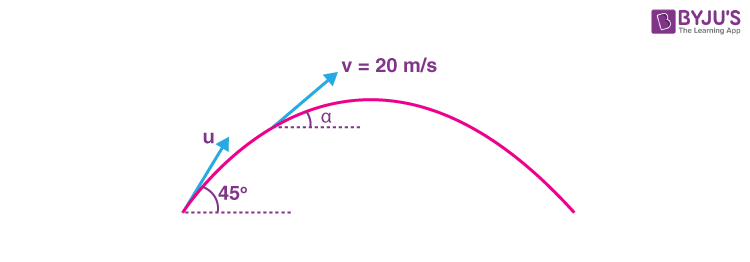v cosα = u cos45° …(i)

& v sinα = usin45° – gt …(ii)

Solve for u we get

$$\begin{array}{l}u =20\sqrt{2} ~\textup{m/s}\end{array}$$
$$\begin{array}{l}\Rightarrow H=\frac{u^2sin^2 45^\circ}{20}=20~\textup{m}\end{array}$$

2. An antenna is placed in a dielectric medium of dielectric constant 6.25. If the maximum size of that antenna is 5.0 mm, it can radiate a signal of minimum frequency of __________GHz.

(Given μr = 1 for dielectric medium)

Sol. We know that v = fλ

Putting the values,

$$\begin{array}{l}\dfrac{3\times10^8} {\sqrt{6.25}}=f\times20\times 10^{-3}\end{array}$$

f = 6 × 109 Hz

3. A potentiometer wire of length 10 m and resistance 20 Ω is connected in series with a 25 V battery and an external resistance 30 Ω. A cell of emf E in secondary circuit is balanced by 250 cm long potentiometer wire. The value of E (in volt) is x/10. The value of x is ___.

Sol.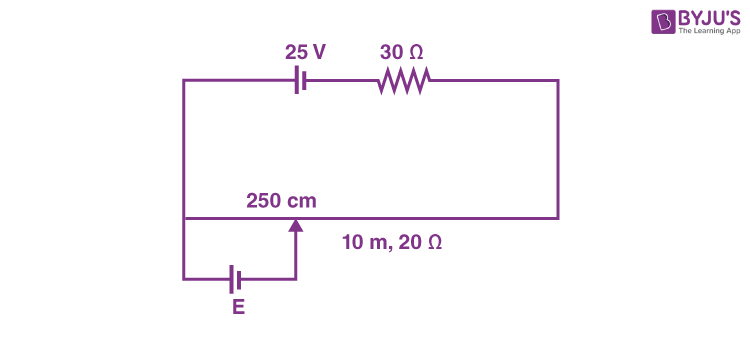$$\begin{array}{l}\therefore E=I\times\left (\frac{20}{4}\right)=\frac{25}{(30+20)}\times \left(\frac{20}{4}\right)\end{array}$$

$$\begin{array}{l}=\frac{1}{2}\times5=2.5~\textup{volts}\end{array}$$
$$\begin{array}{l}=\frac{25}{10}~\textup{volts}\end{array}$$

4. Two travelling waves of equal amplitudes and equal frequencies move in opposite directions along a string. They interfere to produce a stationary wave whose equation is given by

$$\begin{array}{l}y=\left(10\cos \pi x \sin \frac{2\pi t}{T}\right)\textup{cm}\end{array}$$

The amplitude of the particle at

$$\begin{array}{l}x=\frac{4}{3}~\textup{cm}\end{array}$$
will be __________ cm.

Sol.

$$\begin{array}{l}A=\left|10~cos~(\pi x)\right|\end{array}$$
$$\begin{array}{l}At x=\frac{4}{3}\end{array}$$
$$\begin{array}{l}A=\left|10~\textup{cos}\left(\pi\times\frac{4}{3}\right)\right|\end{array}$$
= |– 5 cm|

∴ Amp = 5 cm

5. In the given circuit, the value of current IL will be __________ mA. (When RL = 1 kΩ)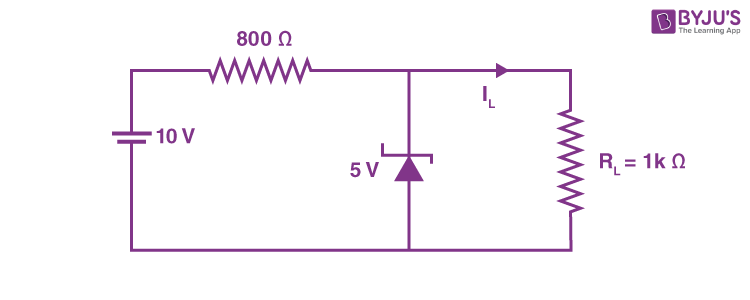Sol. VL = 5 V as VZ = 5 V

$$\begin{array}{l}\therefore I_L=\frac{V_L}{R_L}=\frac{5}{10_3}=5mA\end{array}$$

6. A sample contains 10–2 kg each of two substances A and B with half lives 4 s and 8 s respectively. The ratio of their atomic weights is 1 : 2. The ratio of the amounts of A and B after 16 s is x/100. The value of x is ________.

Sol.

$$\begin{array}{l}N_1=\frac{\left(\frac{10^{-2}}{1}\right)}{2^4}\end{array}$$
$$\begin{array}{l}N_2=\frac{\left(\frac{10^{-2}}{2}\right)}{2^2}\end{array}$$
$$\begin{array}{l}\Rightarrow\frac{N_1}{N_2}=\frac{1}{2}\end{array}$$
∴ Mass ratio of A and B,

$$\begin{array}{l}\frac{m_1}{m_2}=\frac{N_1}{N_2}\times\left(\frac{M_1}{M_2} \right)\end{array}$$
$$\begin{array}{l}=\frac{1}{2}\times\left(\frac{1}{2}\right )\end{array}$$
$$\begin{array}{l}=\frac{1}{4}\end{array}$$
$$\begin{array}{l}=\frac{25}{100}\end{array}$$

x = 25

7. A ray of light is incident at an angle of incidence 60º on the glass slab of refractive index √3. After refraction, the light ray emerges out from other parallel faces and lateral shift between incident ray and emergent ray is 4√3 cm. The thickness of the glass slab is ______ cm.

Sol.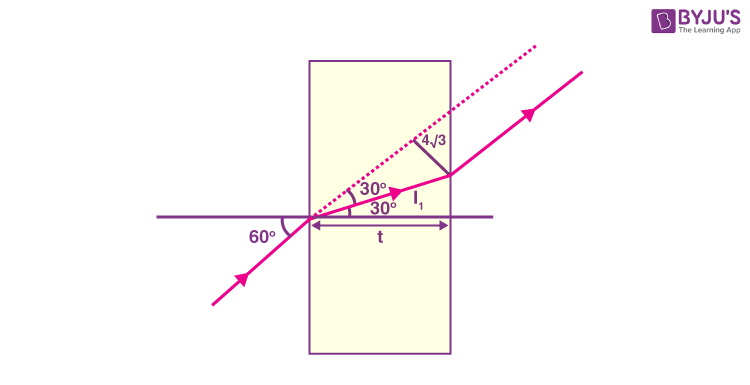$$\begin{array}{l}1\times \textup{sin}~60^{\circ}=\sqrt{3}\times \textup{sin}~ r\end{array}$$

r = 30º

$$\begin{array}{l}\therefore I_1=4\sqrt{3}\times2\end{array}$$
$$\begin{array}{l}=8\sqrt{3}~\textup{cm}\end{array}$$

∴ Thickness, t = l1 cos30º

$$\begin{array}{l}=8\sqrt{3}\times\frac{\sqrt{3}}{2}\end{array}$$

= 4 × 3

= 12 cm

8. A circular coil of 1000 turns each with area 1 m2 is rotated about its vertical diameter at the rate of one revolution per second in a uniform horizontal magnetic field of 0.07 T. The maximum voltage generation will be _______ V.

Sol. Vmax = NABω

= 1000 × 1 × 0.07 × (2π × 1)

≃ 440volts

9. A monoatomic gas performs a work of Q/4where Q is the heat supplied to it. The molar heat capacity of the gas will be ____ R during this transformation. Where R is the gas constant.

Sol. By 1st law,

$$\begin{array}{l}\Delta U=\Delta Q~-~\frac{\Delta Q}{4}=\frac{3}{4}\Delta Q \end{array}$$
$$\begin{array}{l}\Rightarrow nC_v \Delta T =\frac{3}{4}nC\Delta T\end{array}$$
$$\begin{array}{l}\Rightarrow C=\frac{4C_v}{3}=2R\end{array}$$

10. In an experiment to verify Newton’s law of cooling, a graph is plotted between, the temperature difference (ΔT) of the water and surroundings and time as shown in figure. The initial temperature of water is taken as 80ºC. The value of t2 as mentioned in the graph will be ______.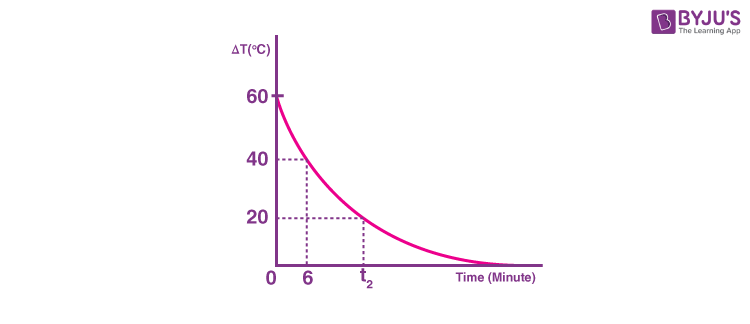Sol. Temperature of surrounding = 20ºC

For 0 → 6 minutes, average temp. = 70ºC

→ Rate of cooling 70ºC – 20ºC = 50ºC

For 6 → t2 minutes, average temp. = 50ºC

→ Rate of cooling 30ºC

$$\begin{array}{l}\Rightarrow t_2-6=\frac{5}{3} (6 \text{ minutes})\end{array}$$

t2 = 16 minutes